SRM vs SynRM performance - basic calculations

Great, you’re back!

I mean, you’re here for the second piece of the SRM-vs-SynRM post series, right? Right?

Well in any case, here’s what we’ll have today. We’ll look into some approaches for comparing the two motor topologies. Let me warn you – there won’t be any definite conclusions, as so much depends on the application. Instead, we’ll simply cover some general methodologies that can then be applied to the problem at hand.

For that purpose, now would be a good time to take a look at the older post series on different torque computation methods. As you hopefully remember, there are more than enough approaches, each more suitable for some problems than others. And nowhere else is this more evident than with SRMs and SynRMs.

Let’s begin with the SRM.

### SRM modelling basics

To compute the torque of a SRM, reluctance network and inductance-based approaches are the easiest choice.

Let’s begin by considering only one stator tooth and rotor pole. The inductance of the stator coil is roughly proportional to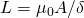,

where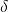is the airgap length and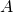is the overlapping area of the tooth and pole. Next, assuming a fixed current, we can write the instantaneous power balance as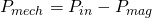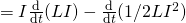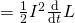.

In other words, the input power (after resistive losses) = current times induced voltage, is spent on mechanical work plus increasing the energy stored in the magnetic field.

By writing open the derivative of the inductance with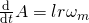,

and dividing the power by the speed to get torque, we get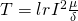.

##### Alternative way

Now, for the sake of learning and confidence both, let’s approach this problem from another angle, and try to get the same result. This time, let’s use a super-simple reluctance network. For the same tooth-pole pair, we get for the torque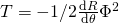,

where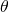is the rotor angle and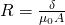is the reluctance of the airgap. By writing the derivative as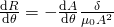,

and noting that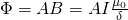,

we get for the torque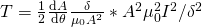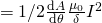.

By substituting in the earlier definition for the derivative of, we indeed get the same result as with the derivative approach!

Sidenote: These two approaches represent the two sides of the virtual work principle: co-energy and energy, respectively. The reluctance network method is a straightforward application of the energy method: there’s no power coming into the system, so any change in the stored energy must be reflected in mechanical work. By contrast, the inductance method utilizes the concept of coenergy: a combination of released magnetic energy and input power.

##### Total torque

Of course, the example above was highly simplistic.

First of all, a single coil cannot of course produce torque all the time. Once the rotor pole is fully aligned, the coil has to be quickly switched off until the pole has passed and the next one is beginning to align, lest braking torque be generated.

As a consequence, a single coil can only produce torque half of the time, tops. Often, even less than that.

Furthermore, analysing only a single coil at a time might be too simplistic. Meaning, the airgap flux density over a pole might enjoy a more complex relationship than current per gap length. Indeed, the numbers of stator and rotor poles and the winding configuration can all influence the results.

Meaning, you’ll either have to define the mutual inductances between phases, or use a little more complex reluctance network.

But let’s finally move on to the SynRM.

### SynRM modelling basics

…aaand get stuck almost immediately. The thing is, SynRMs are complicated. They have a complex rotoe geometry plus heavy saturation inside it, resulting in a hard-to-predict airgap flux density.

For that reason, finite element analysis is your best bet.

A largish reluctance network is your second-best choice. We’re talking at least one element for each flux guide, one or a few for each barrier, one for each rip, and maybe one in the airgap for each stator tooth.

Obviously, neither of these approaches is particularly good for quick analysis.

So, a rough approximation based on typical performance levels will have to do. Here’s what I suggest.

##### Approximations

As you probably know, a SynRM has a salient rotor – it “conducts” flux better in one direction than others. Now, behaviour in this easy axis – d-axis – is quite easy to approximate. Indeed, using the standard permeance model, with the flux density being the magnetomotive force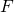multiplied by the (specific) permeance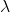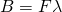is probably good enough.

Now, the permeance in general depends on the angular position in the airgap. For simplicity, let’s just assume it varies sinusoidally (and that we have a two-pole machine), so we have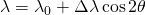.

(Mind you, the symbolnow signifies the angular position in the airgap, rather than rotor position.)

We know that its maximum is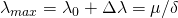.

Furthermore, from typical behaviour we know its minimum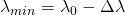is around 1/5 to 1/8 of the maximum. This gives us two equations for the two unknowns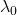and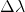, letting us solve both.

Now, once we have the expression for the permeance, we can use it to compute the torque. First, we get the airgap flux density from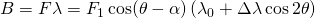.

The angle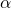denotes the angle difference between the rotor d-axis and the stator field. I like to call it the load angle, although some people probably disagree on that. But I digress.

By using a little trigonometric magic, we can expand the flux density into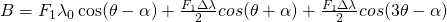.

This flux density can then produce torque by interacting with the surface (or linear) current density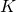,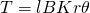.

In case you’ve forgotten,is equal to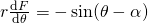.

Of the three flux density terms, only the middle one can produce net torque. So, we can expand the product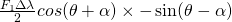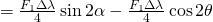.

And again, only the first term here produces a non-zero net torque when integrated over the airgap.

##### Implications

From the formula above, we see that for given, we get the maximum torque when there’s a 45 degree angle between the mmf and the rotor axis.

However, if we increase the angle, the maximum flux density in the airgap goes down. On one hand, the flux density is a hard constraint – it’s tricky to have much more than 1.8 T or 2 T anywhere, thanks to the saturation of iron. And on the other hand, the torque is still proportional to the product of the flux density and current.

Meaning, if we increase the rotor angle, we can also increase the surface current density to maintain the flux density. And once you multiply-integrate the flux and current densities, you may well end up with a larger torque. But only up to a point – the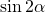term in the torque equation will start bringing the torque down once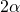gets close enough to 90 degrees.

The following example should illustrate the point. Let’s say our “permeance” varies between 1 and 0.2 like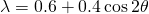.

A saliency ratio of 5, in other words. This is also illustrated in the figure below.

Now, let’s say our mmf is also sinusoidal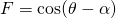as earlier.

Now, we multiplyandto get the flux density, shown below for different load angles. As you can see, the maximum flux density gets smaller as the load angle is increased.

Finally, let’s compute the torque by integrating the product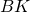around the airgap (where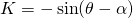). Below, you can see the torque curves as a function of the load angle, in two cases. The first one, the pure sinusoid, is the textbook version with a fixed current. The second one, on the other hand, has the current increased with the load angle, so that the maximum flux density is maintained at 1 unit.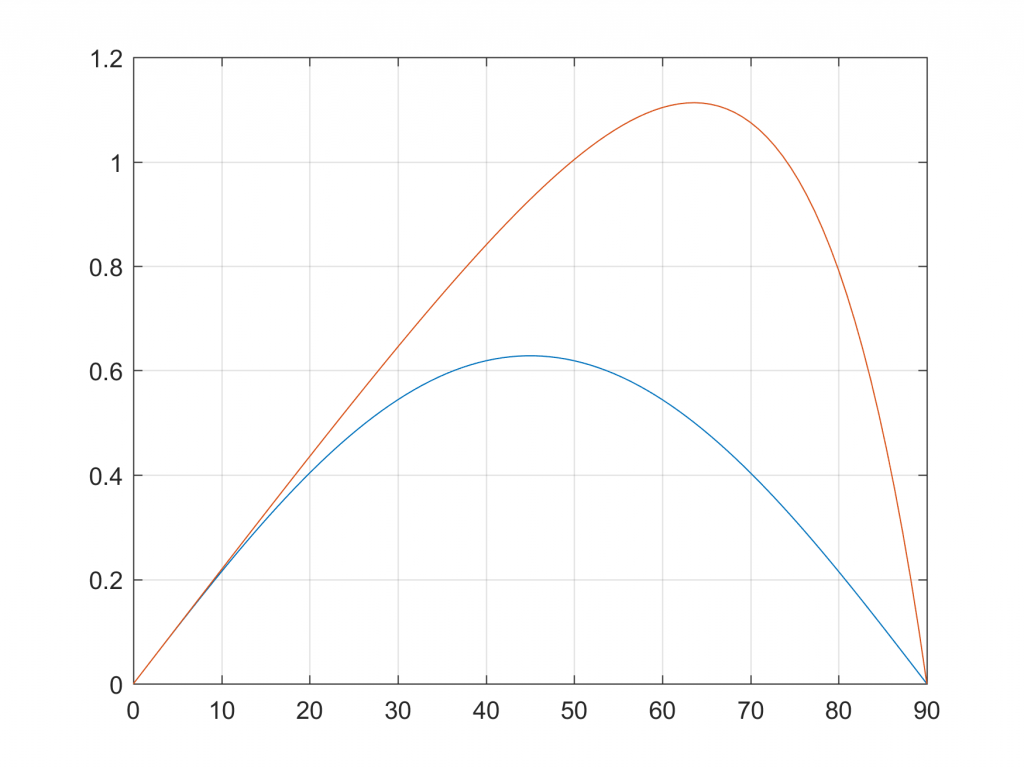Torque curves as a function of the load angle, with a constant current density (blue curve) and with a fixed maximum flux density (red curve).

As can be seen, almost twice as large maximum torque can be reached with the latter approach. Furthermore, the maximum is reached at approximate 63 degrees load angle – significantly larger than the textbook 45 degrees!

In reality, however, there are some complications. Increasing the current of course increases the losses – an important factor to consider. Different leakage fields can also be increased, and influence the results. And finally, our sinusoidal permeance function is very much an approximation. Reality is, again, much more complex.

### Conclusion

Phew, there you have the very basics. The beginning of the basics, let’s say. You now have some rough tools to calculate the torque of a switched and synchronous reluctance motor, from the airgap length and the total current in a slot, basically.

Next time, we’ll see how these numbers translate to the overall size of the machine, and maybe also take a brief look at some thermal aspects.

Need help with electric motor design or design software? Let's get in touch - satisfaction guaranteed!SRM vs SynRM Performance Basics